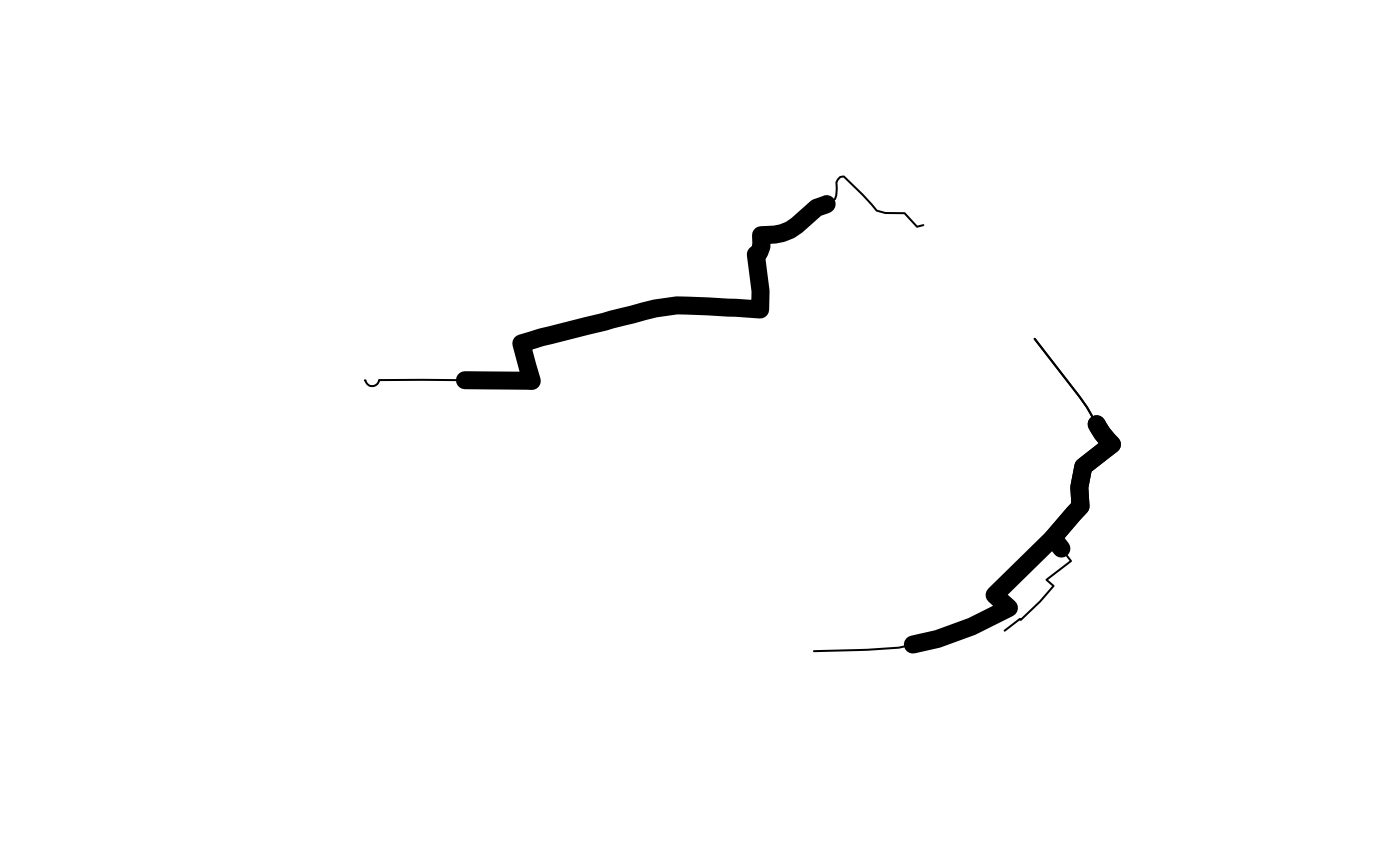Takes lines and removes the start and end point, to a distance determined by the user.

## Usage

``geo_toptail(l, toptail_dist, ...)``

## Arguments

l

An `sf` object representing lines

toptail_dist

The distance (in metres) to top and tail the line by. Can either be a single value or a vector of the same length as the SpatialLines object.

...

Arguments passed to `sf::st_buffer()`

## Details

Note: see the function `toptailgs()` in stplanr v0.8.5 for an implementation that uses the geosphere package.

Other lines: `angle_diff()`, `is_linepoint()`, `line2df()`, `line2points()`, `line_bearing()`, `line_breakup()`, `line_midpoint()`, `line_segment1()`, `line_segment()`, `line_via()`, `mats2line()`, `n_segments()`, `n_vertices()`, `onewaygeo()`, `points2line()`, `toptail_buff()`

## Examples

``````lib_versions <- sf::sf_extSoftVersion()
lib_versions
#>           GEOS           GDAL         proj.4 GDAL_with_GEOS     USE_PROJ_H
#>       "3.10.2"        "3.4.1"        "8.2.1"         "true"         "true"
#>           PROJ
#>        "8.2.1"
# dont test due to issues with sp classes on some set-ups
if (lib_versions >= "6.3.1") {
l <- routes_fast_sf[2:4, ]
l_top_tail <- geo_toptail(l, 300)
l_top_tail
plot(sf::st_geometry(l_top_tail))
plot(sf::st_geometry(geo_toptail(l, 600)), lwd = 9, add = TRUE)
}``````